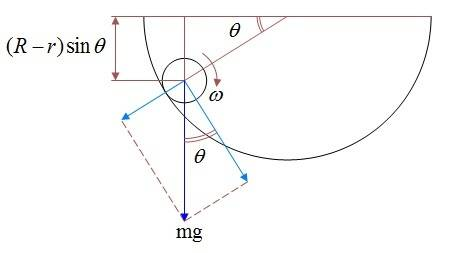# Ball rolls without sliding in a concave bowl

• Karol
In summary, the problem involves a ball of mass m and radius r rolling without sliding in a bowl of radius R. The angular velocity of the ball, ω, is expressed as a function of the angle θ using the equations of moment of inertia, Sheiner's theorem, kinetic energy of a rigid body, and torque and angular acceleration. The normal force and friction force are also expressed as a function of θ using the equations of centripetal force and torque. However, it is important to note that the concept of centripetal force should be replaced with that of centripetal acceleration, and that the signs and units should be carefully considered to ensure accuracy.

## Homework Statement

Ball of mass m and radius r rolls without sliding in a bowl of radius R.
Express it's angular velocity ω as a function of the angle θ.
What are the normal force and friction force as a function of θ.## Homework Equations

Moment of inertia of a solid ball round it's center: ##I_c=\frac{2}{5}mr^2##
Sheteiner's theorem: ##I_A=I_c+mr^2##
Kinetic energy of a rigid body: ##E=\frac{1}{2}I\omega^2##
Centripetal force: ##F=mr\omega^2##
Torque and angular acceleration: ##M=I\alpha=I\dot\omega##

## The Attempt at a Solution

The descending in height is transferred to kinetic energy:
$$mg(R-r)\sin\theta=\frac{1}{2}\left( \frac{2}{5}mr^2+mr^2 \right)\omega^2\;\rightarrow\; \omega^2=\frac{10}{7}\frac{R-r}{r^2}g\sin\theta$$
$$\omega=\left( \frac{1}{r}\sqrt{\frac{10}{7}g(R-r)} \right) \cdot (\sin\theta)^{\frac{1}{2}}$$
$$\dot\omega=\left( \frac{1}{2r}\sqrt{\frac{10}{7}g(R-r)} \right) \frac{\cos\theta}{\sqrt{\sin\theta}}$$
The normal force is the substarction of the weight's radial component and the centripetal one:
$$F=mg\sin\theta-\frac{1}{2}I\omega^2=mg\sin\theta-\frac{10}{7}\frac{R-r}{r^2}g\sin\theta=mg\sin\theta\left( \frac{10}{7}\frac{R-r}{r}+1 \right)$$
The friction force:
$$M=f\cdot r=I_c\dot\omega\;\rightarrow\; f=\frac{2}{5}mr\cdot\left( \frac{1}{2r}\sqrt{\frac{10}{7}g(R-r)} \right) \frac{\cos\theta}{\sqrt{\sin\theta}}$$

I don't see the connection between the centripetal force and ##-\frac 12 I\omega^2##. I count four things wrong there.

$$F=mg\sin\theta-mr\omega^2=mg\sin\theta-\frac{2}{5}mr^2\cdot \frac{10}{7}\frac{R-r}{r^2}g\sin\theta=mg\sin\theta\left(1+ \frac{4}{7}\frac{R-r}{r} \right)$$

Karol said:
##-mr\omega^2##
That fixed two problems, two to go.
Remember that the centripetal force is a component of the resultant of the actual forces. In fact, it is better to renounce the concept of centripetal force and think instead in terms of centripetal acceleration.
To make sure the signs are right, get into the habit of writing such equations in the standard Fnet=ma form, where the net force is the resultant of the actual forces.
The other problem concerns the (angular) velocity and radius of the centripetal acceleration. Where is the centre of curvature of the trajectory of the ball's centre?

$$F=m(R-r)\omega^2-mg\sin\theta=m(R-r)\cdot \frac{10}{7}\frac{R-r}{r^2}g\sin\theta-mg\sin\theta=mg\sin\theta\left(1+ \frac{10}{7}\left( \frac{R-r}{r} \right)^2 \right)$$

Still not right.
I assume you are taking g to have a positive value. If F is the normal force, what is the net radial force on the ball?
Also, you are confusing ##\omega## with ##\dot\theta##.

$$F=m\frac{v^2}{R-r}+mg\sin\theta=\frac{m}{R-r}\omega^2r^2+mg\sin\theta=\frac{m}{R-r}\cdot \frac{10}{7}\frac{R-r}{r^2}g\sin\theta r^2+mg\sin\theta=mg\sin\theta\left(1+ \frac{10}{7} \right)$$

Karol said:
$$F=m\frac{v^2}{R-r}+mg\sin\theta=\frac{m}{R-r}\omega^2r^2+mg\sin\theta=\frac{m}{R-r}\cdot \frac{10}{7}\frac{R-r}{r^2}g\sin\theta r^2+mg\sin\theta=mg\sin\theta\left(1+ \frac{10}{7} \right)$$
Yes, that looks right.

Thank you Haruspex